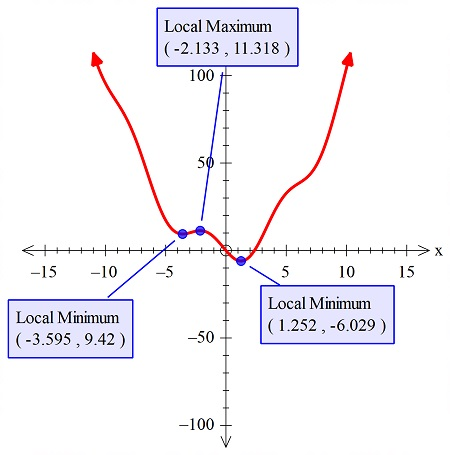# Find all extreme values of f(x) = x^2-8sinx .

## Question:

Find all extreme values of f(x) = {eq}x^2-8sinx {/eq}.

## Finding Local Extrema:

It can be noted that the graphing calculator is used to graph the function, that has a trigonometric and the algebraic expressions together. Now the extremes can easily be labeled and shown with the help of the graphing calculator.

So find the extremes of the curve, we need to find the graph of the function.

So the figure below is showing the graph:Now, the...

Become a Study.com member to unlock this answer! Create your account